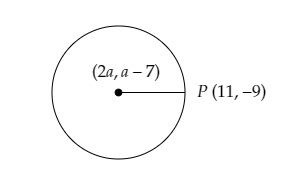# The centre of a circle is $(2a, a â€“ 7)$. Find the values of $a$ if the circle passes through the point $(11, -9)$ and has diameter $10\sqrt2$ units.

Given:

The centre of a circle is $(2a, a – 7)$.

To do:

We have to find the values of $a$ if the circle passes through the point $(11, -9)$ and has diameter $10\sqrt2$ units.

Solution:From the figure,

Radius of the circle $=$ Distance between the centre $C (2a, a-7)$ and the point $P (11, -9)$

We know that,

The distance between two points $(x_{1}, y_{1})$ and $(x_{2}, y_{2})=\sqrt{(x_{2}-x_{1})^{2}+(y_{2}-y_{1})^{2}}$

Radius of the circle $=\sqrt{(11-2a)^2+(-9-a+7)^2}$

$=\sqrt{(11-2a)^2+(2+a)^2}$......(i)

The length of the diameter $=10 \sqrt{2}$ units. This implies,

The length of the radius $=\frac{\text { Length of diameter }}{2}$

$=\frac{10 \sqrt{2}}{2}=5 \sqrt{2}$

Therefore,

$5 \sqrt{2}=\sqrt{(11-2 a)^{2}+(-2-a)^{2}}$

Squaring on both sides, we get,

$50=(11-2 a)^{2}+(2+a)^{2}$

$\Rightarrow 50=121+4 a^{2}-44 a+4+a^{2}+4 a$

$\Rightarrow 5 a^{2}-40 a+75=0$

$\Rightarrow a^{2}-8 a+15=0$

$\Rightarrow a^{2}-5 a-3 a+15=0$

$\Rightarrow a(a-5)-3(a-5)=0$

$\Rightarrow(a-5)(a-3)=0$

$\therefore a=3,5$

Hence, the required values of $a$ are 5 and 3.

Updated on: 10-Oct-2022

75 Views